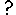#Condition

A condition model element is used to specify whether a submodel, or a potential instance of a multiple-instance submodel, actually exists.

## How to add a Condition symbol

The condition symbol only has meaning inside a submodel. Therefore, you should make the submodel first, then add the condition symbol to it. This is not strictly necessary: you can add the condition symbol first, then construct a submodel around it, but it is better practice to construct the submodel first.

## Rules

• A condition element only has use within a submodel (since it specifies which potential instances exist). It should not appear in a population or per-record submodel because these have their own means of creating and destroying instances.
• The expression in the equation dialogue box for a condition element is a Boolean expression: that is, it returns the value "true" or "false". This normally takes the form of some sort of comparison, using the conditional operators such as >, <, >= etc, combined with logical operators such as "and" and "or". However, conditions of the form "index(1) is <expr>" or "any(index(1) is <list_expr>)" are also allowed; see one-sided relation enumeration.
• A condition may have influences to it from anywhere in the model. It cannot have influences from it, since either its value is "true" or its submodel instance does not exist.
• If a condition is inside a simple submodel, then that submodel either exists or not, depending on the result of evaluating the condition's expression.
• If a condition is inside a fixed-membership submodel, then each instance of the submodel may exist or not, depending on the condition's expression (which would make use of the built-in function index to refer to particular instances).
• If the condition model element is inside an association submodel, then the relation exists between a particular pair of object instances only if the condition's expression evaluates to "true" for that pair. The condition's equation can use values from base instances in particular roles in order to set the association to exist only if the base instances' values match in a certain way.